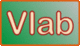online_headloss_venturi_meter:  Head loss using a Venturi meterA Venturi meter
 ν = f(T) ReD2 = 4Q/(πD2ν) K = f(ReD2, D2/D1) A2 = (π/4) D22 hf = [Q/(KA2)]2/(2g) h = hf/[(ρ2/ρ1) - 1]

[SI units: Q in m3/s; D in m; T in °C; h in m]
[U.S. units: Q in cfs; D in ft; T in °F; h in ft]

INPUT DATA:

Select:

 SI units (metric) U.S. Customary units

Discharge Q :

Diameter D1 :

Diameter D2 :

Temperature T :

Ratio ρ21 :

[Leave blank to set mercury manometer
as default ρ21 = 13.6]

OUTPUT:

hρ2 =  0

hρ1 =  0

Your request was processed at  08:30:05 am on July 5th, 2022   [ 220705  08:30:05 ].

Thank you for running online_discharge_venturi_meter.